# Defining an Axis SystemThis task explains how to define a new three-axis system locally. There are two ways of defining it: either by selecting geometry or by entering coordinates.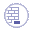Open the PowerCopyStart1.CATPart document.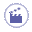1. Select Insert > Axis System from the menu bar or click Axis System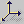.

The Axis System Definition dialog box is displayed.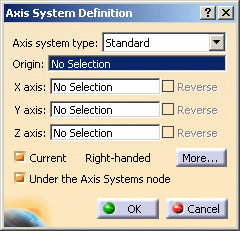An axis system is composed of an origin point and three orthogonal axes. For instance, you can start by selecting the vertex as shown to position the origin of the axis system you wish to create. The application then computes the remaining coordinates. Both computed axes are then parallel to those of the current system. The axis system looks like this: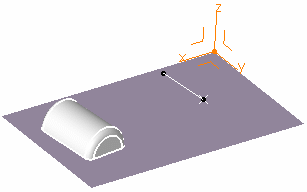It can be right or left-handed. This information is displayed within the Axis System Definition dialog box.
You can choose from different types of axis system:
• Standard: defined by a point of origin and three orthogonal directions.
If an axis system is selected before launching the command, the new axis system is a copy of the pre-selected axis system. Moreover, if the compass is attached to the 3D geometry, the new axis system orientations are the same as the compass'. Otherwise, the new axis system orientations are as per the current axis system's.

Here only the point was selected and nothing specified for the axes.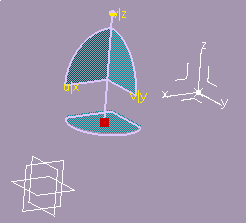• Axis rotation: defined as a standard axis system and a angle computed from a selected reference.

Here the Y axis was set to the standard axis system Y axis, and a 15 degrees angle was set in relation to an edge parallel to the X axis.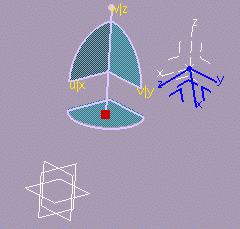• Euler angles: defined by three angle values as follows:

Angle 1= (X, N)

a rotation about Z transforming vector X into vector N.

Angle 2= (Z, W)

a rotation about vector N transforming vector Z into vector W.

Angle 3= (N, U)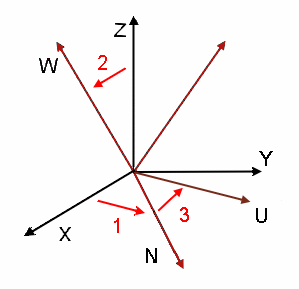2. Select the point as shown to position the origin of the axis system you wish to create. The application then computes the remaining coordinates. Both computed axes are then parallel to those of the current system. The axis system looks like this: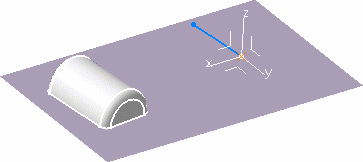Instead of selecting the geometry to define the origin point, you can use one of the following contextual commands available from the Origin field: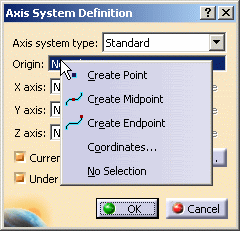Create Point: for more information, refer to Points Coordinates: for more information, refer to Points Create Midpoint: the origin point is the midpoint detected by the application after selection of a geometrical element.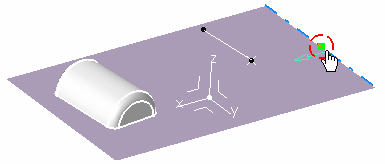Create Endpoint: the origin point is the endpoint detected by the application after selection of a geometrical element.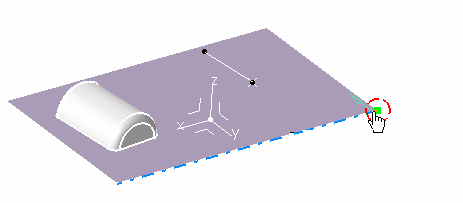3. If you are not satisfied with x axis, for instance click the X Axis field and select a line to define a new direction for x axis.

 The x axis becomes collinear with this line.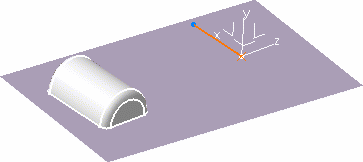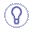It can be a line created along the surface edge, for example, using the Create Line contextual menu on the selection field, and selecting two surface vertices. Similarly you can create points, and planes. You can also select the Rotation... contextual menu, and enter an angle value in the X Axis Rotation dialog box.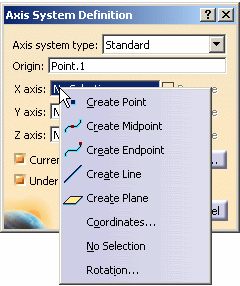4. Click the y axis in the geometry to reverse it.

 Checking Reverse next to the Y Axis field reverses its direction too.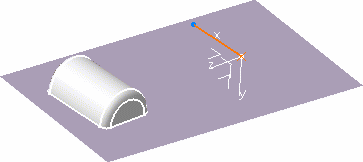5. You can also define axes through coordinates. Right-click the Z Axis field and select the Coordinates... contextual command. The Z Axis dialog box appears.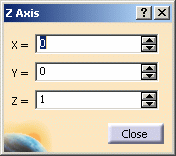6. Key in X = -1, retain the Y and Z coordinates, and click Close.

 The axis system is modified accordingly, and is now left-handed.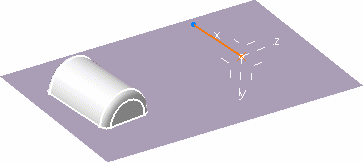7. Click More... to display the More... dialog box.The first row contains the coordinates of the origin point. The coordinates of X axis are displayed in the second row. The coordinates of Y and Z axis are displayed in the third and fourth row respectively. If no value is selected, the new axis system matches the current one. If the origin is selected, the new axis system origin is set to the origin. The first specified axis defines the corresponding axis of new axis system. i.e., if the x-axis is specified by Line.1, then the x-axis of new system is a vector along Line.1. The second specified axis defines the plane between the corresponding first and second axes of the new axis system. i.e., if the z-axis is specified by Line.2, then the xz plane is defined by the plane between vectors along Line.1 and Line.2. The third specified axis defines the orientation of the corresponding axis of new axis system. i.e., if the y-axis is specified by Line.3, then Line.3 defines which side of the xz plane the y-axis of new system lies. The order of selection of the axes is important: to change the order, select the No Selection contextual item on the appropriate axes. For instance, if the axes have been selected in the order x, y, z and you wish to change the order to x, z, y, you must select the No Selection contextual item on y, and select it again.
8. Uncheck Current if you do not want to set your axis as the reference. The absolute axis at the bottom right of the document then becomes the current three axis system.

9. Uncheck Under the Axis Systems node if you do not want the axis system to be created within the Axis system node in the specification tree.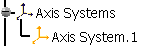It will be created either in the current geometrical set or right after the current object in an ordered geometrical set. In this case, the axis system becomes the new current object.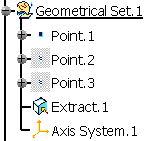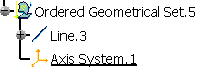10. Click OK.

 The axis system is created. When it is set as current, it is highlighted in the specification tree.
11. Right-click Axis System.1 from the specification tree and select the Axis System.1 object > Set As Current contextual command. Axis System.1 is now current. You can then select one of its plane, to define a sketch plane for example.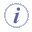• You can change the location of the axis system and put it in a geometrical set.
To do so, select it in the specification tree, right-click and select Axis System.1 object > Change Geometrical Set. Choose the destination of the axis system using the drop-down list. Refer to the Managing Geometrical Sets chapter to have more information.
• If you create a point using the coordinates method and an axis system is already defined and set as current, the point's coordinates are defined according to current the axis system. As a consequence, the point's coordinates are not displayed in the specification tree.
• You can contextually retrieve the current local axis direction. Refer to the Stacking Commands chapter to have more information.
• You can use the Shift key while creating the axis system to select the implicit elements that belong to the axis system. Refer to the Selecting Implicit Elements chapter to have more information.
• There is an associativity between the feature being created and the current local axis system. Therefore when the local axis system is updated after a modification, all features based on the axis direction are updated as well.
• Local axes are fixed. If you wish to constrain them, you need to isolate them (using Isolate contextual command) before setting constraints otherwise you would obtain over-constrained systems.
• The display mode of the axes is different depending on whether the three-axis system is right-handed or left-handed and current or not.  Three-Axis System Current Axis Display Mode right-handed yes solid right-handed no dashed left-handed yes dotted left-handed no dot-dashed

## Editing an Axis System

 You can edit your axis system by double-clicking it and entering new values in the dialog box that appears. You can also use the compass to edit your axis system. Note that editing the geometrical elements selected for defining the axes or the origin point affects the definition of the axis system accordingly. Right-clicking Axis System.X object in the specification tree lets you access the following contextual commands: Definition...: redefines the axis system Isolate: sets the axis system apart from the geometry Set As Current/Set As Not Current: defines whether the axis system is the reference or not.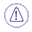Under the Axis Systems node is not available when editing an axis system.